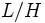# Finite normal implies amalgam-characteristic

This article gives the statement and possibly, proof, of an implication relation between two subgroup properties. That is, it states that every subgroup satisfying the first subgroup property (i.e., finite normal subgroup) must also satisfy the second subgroup property (i.e., amalgam-characteristic subgroup)
View all subgroup property implications | View all subgroup property non-implications
Get more facts about finite normal subgroup|Get more facts about amalgam-characteristic subgroup
This article gives the statement and possibly, proof, of an implication relation between two group properties. That is, it states that every group satisfying the first group property (i.e., finite group) must also satisfy the second group property (i.e., always amalgam-characteristic group)
View all group property implications | View all group property non-implications
Get more facts about finite group|Get more facts about always amalgam-characteristic group

## Statement

### Verbal statement

Any finite normal subgroup (i.e., a normal subgroup that is finite as a group) is an amalgam-characteristic subgroup.

Equivalently, a finite group is an always amalgam-characteristic group: it is an amalgam-characteristic subgroup in any group in which it is a normal subgroup.

### Statement with symbols

Suppose$H$ is a finite normal subgroup of a group$G$. Then,$H$ is a characteristic subgroup inside the amalgam$K := G *_H G$.

## Proof

Given: A group$G$, a finite normal subgroup$H$ of$G$.$K := G *_H G$.

To prove:$H$ is a characteristic subgroup in$L$.

Proof:

1. By fact (1),$K/H \cong G/H * G/H$.
2.$K/H$ has no nontrivial finite normal subgroup: By fact (2), if$H$ is a proper subgroup of$G$, then$L/H$ has no nontrivial finite normal subgroup. If$H = G$, then$L/H$ is trivial and hence has no nontrivial finite normal subgroup.
3.$H$ is a finite normal subgroup of$K$: Since$H$ is normal in both copies of$G$, it is normal in$K$. Also,$H$ is finite.
4.$H$ is the unique largest finite normal subgroup of$K$: Suppose$M$ is a finite normal subgroup of$K$. Then, by fact (4), the image of$M$ in the quotient map$K \to K/H$ is a normal subgroup of$K/H$. Also, since$M$ is finite, its image is finite. In step (2), we concluded that$K/H$ has no nontrivial finite normal subgroup. Thus, the image of$M$ is trivial, so$M \le H$.
5.$H$ is characteristic in$K$: This follows since it is the unique largest finite normal subgroup of$K$.